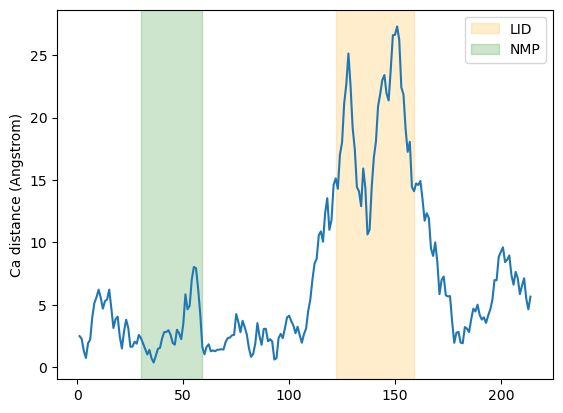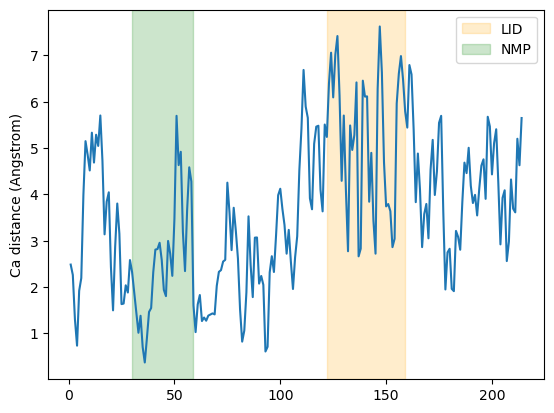# Atom-wise distances between matching AtomGroups

Here we compare the distances between alpha-carbons of the enzyme adenylate kinase in its open and closed conformations. distances.dist can be used to calculate distances between atom groups with the same number of atoms within them.

Last updated: December 2022 with MDAnalysis 2.4.0-dev0

Minimum version of MDAnalysis: 0.19.0

Packages required:

Optional packages for visualisation:

:

import MDAnalysis as mda
from MDAnalysis.tests.datafiles import PDB_small, PDB_closed
from MDAnalysis.analysis import distances

import matplotlib.pyplot as plt
%matplotlib inline

import warnings
# suppress some MDAnalysis warnings when writing PDB files
warnings.filterwarnings('ignore')


The test files we will be working with here feature adenylate kinase (AdK), a phosophotransferase enzyme. ([BDPW09]) AdK has three domains:

• CORE

• LID: an ATP-binding domain (residues 122-159)

• NMP: an AMP-binding domain (residues 30-59)

The LID and NMP domains move around the stable CORE as the enzyme transitions between the opened and closed conformations.

:

u1 = mda.Universe(PDB_small)   # open AdK
u2 = mda.Universe(PDB_closed)  # closed AdK


## Calculating the distance between CA atoms

We select the atoms named ‘CA’ of each Universe.

:

ca1 = u1.select_atoms('name CA')
ca2 = u2.select_atoms('name CA')


distances.dist(API docs) returns the residue numbers of both selections given. The offset keyword adds an offset to these residue numbers to help with comparison to each other and other file formats. Here we are happy with our residue numbers, so we use the default offset of 0. (See the documentation of distances.dist for more information.)

:

resids1, resids2, dist = distances.dist(ca1, ca2,
offset=0)  # for residue numbers


### Plotting

Below, we plot the distance over the residue numbers and highlight the LID and NMP domains of the protein. The LID domain in particular moves a significant distance between its opened and closed conformations.

:

plt.plot(resids1, dist)
plt.ylabel('Ca distance (Angstrom)')
plt.axvspan(122, 159, zorder=0, alpha=0.2, color='orange', label='LID')
plt.axvspan(30, 59, zorder=0, alpha=0.2, color='green', label='NMP')
plt.legend()

:

<matplotlib.legend.Legend at 0x7f06d2a3b7c0>## Calculating the distance with periodic boundary conditions

It is common to want to calculate distances with the minimum image convention. To do this, you must pass the unitcell dimensions of the system to the box keyword, even if your Universe has dimensions defined.

This should have the format: [lx, ly, lz, alpha, beta, gamma], where the first three numbers are the box lengths along each axis and the last three are the angles of the box.

:

resids1_box, resids2_box, dist_box = distances.dist(ca1, ca2,
box=[10, 10, 10, 90, 90, 90])


### Plotting

:

plt.plot(resids1_box, dist_box)
plt.ylabel('Ca distance (Angstrom)')
plt.axvspan(122, 159, zorder=0, alpha=0.2, color='orange', label='LID')
plt.axvspan(30, 59, zorder=0, alpha=0.2, color='green', label='NMP')
plt.legend()

:

<matplotlib.legend.Legend at 0x7f06d09bc700>